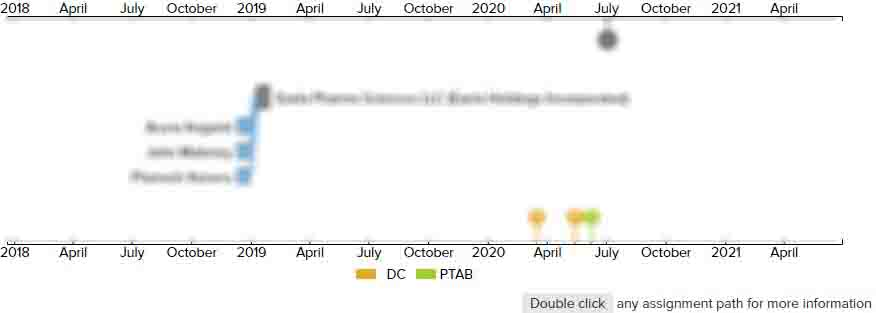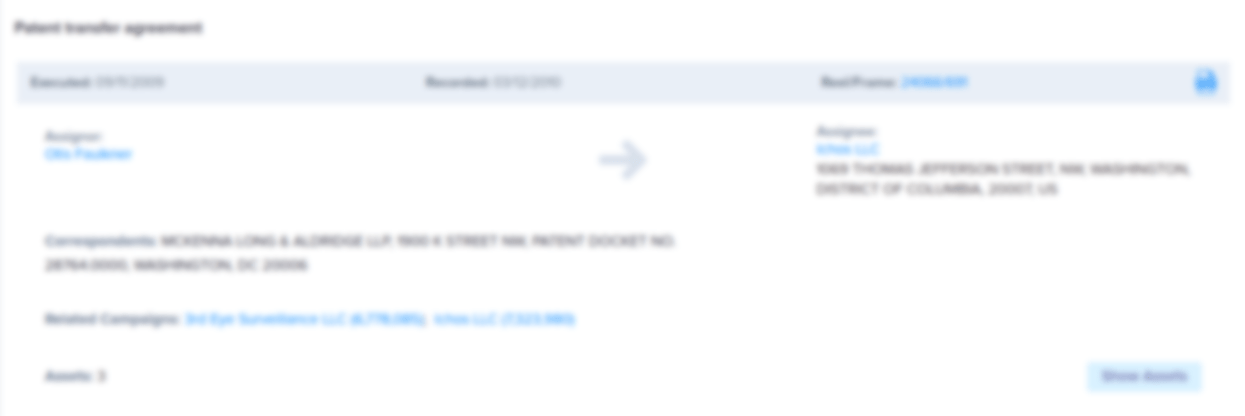# Digital monopulse

• US 5,315,304 A
• Filed: 07/02/1993
• Issued: 05/24/1994
• Est. Priority Date: 07/02/1993
• Status: Expired due to Fees
##### First Claim
Patent Images

1. A digitally formed monopulse system having a transmission/calibration cycle and a receive cycle, said system comprising:

• means for signal transmission to and return signal reception from an environment external to said system;

means for producing signals for external transmission and internal signal processing, said producing means connected to said means for signal transmission and reception, and to said means for internal signal processing;

means for diverting a portion of said signal produced for external transmission to said means for internal signal processing during said transmission/calibration cycle; and

means for internal signal processing comprising four signal processing channels for simultaneous processing of four-quadrant antenna transmit and receive signal information connected to said means for transmission and reception, said signal processing channels for calculating calibration factors reflecting system characteristics during the transmission/calibration cycle of said system, for calculating corrected signals received during the immediately following receive cycle, and for calculating the monopulse information derived therefrom andwherein each of said channels provides in-phase and quadrature signals to a digital signal processor for production of calibration factors, where said in-phase and quadrature signals in one channel are used as the standard for correcting the other three channels to that standard in accordance with the following equations;

space="preserve" listing-type="equation">K(I2)=I(1)/I(2)

space="preserve" listing-type="equation">K(Q2)=Q(1)/Q(2)

space="preserve" listing-type="equation">K(I3)=I(1)/I(3)

space="preserve" listing-type="equation">K(Q3)=Q(1)/Q(3)

space="preserve" listing-type="equation">K(I4)=I(1)/I(4)

space="preserve" listing-type="equation">K(Q4)=Q(1)/Q(4) where K(I2), K(Q2), K(I3), K(Q3), K(I4), K(Q4) are the calibration factors calculated as the ratio of the standard channel I(1) and Q(1) divided by each of the other channels I(2), Q(2), I(3), Q(3), I(4), and Q(4), and where during said receive cycle said means for digital signal processing uses the correction factors calculated during the calibration cycle to calculate the corrected signals received in accordance with the following equations;

space="preserve" listing-type="equation">I'"'"'(1)=I(1)

space="preserve" listing-type="equation">Q'"'"'(1)=Q(1)

space="preserve" listing-type="equation">I'"'"'(2)=I(2)×

K(I2)

space="preserve" listing-type="equation">Q'"'"'(2)=Q(2)×

K(Q2)

space="preserve" listing-type="equation">I'"'"'(3)=I(3)×

K(I3)

space="preserve" listing-type="equation">Q'"'"'(3)=Q(3)×

K(Q3)

space="preserve" listing-type="equation">I'"'"'(4)=I(4)×

K(I4)

space="preserve" listing-type="equation">Q'"'"'(4)=Q(4)×

K(Q4) where the primes are the corrected values then used by said digital signal processing means to calculate the sum (Σ

)

space="preserve" listing-type="equation">Σ

=+ + + 4 where 1, 2, 3, and 4 are the complex I and Q values for each channel, and where said digital signal processing means calculatesthe delta pitch (Δ

p)

space="preserve" listing-type="equation">Δ

p=(1+2)-(3+4) andthe delta yaw (Δ

y)

space="preserve" listing-type="equation">Δ

y=(1+3)-(2+4); and

the delta diagonal (Δ

d)

space="preserve" listing-type="equation">Δ

d=(1+4)-(2+3).

• ##### 1 Assignment
Timeline View
Assignment View×
×